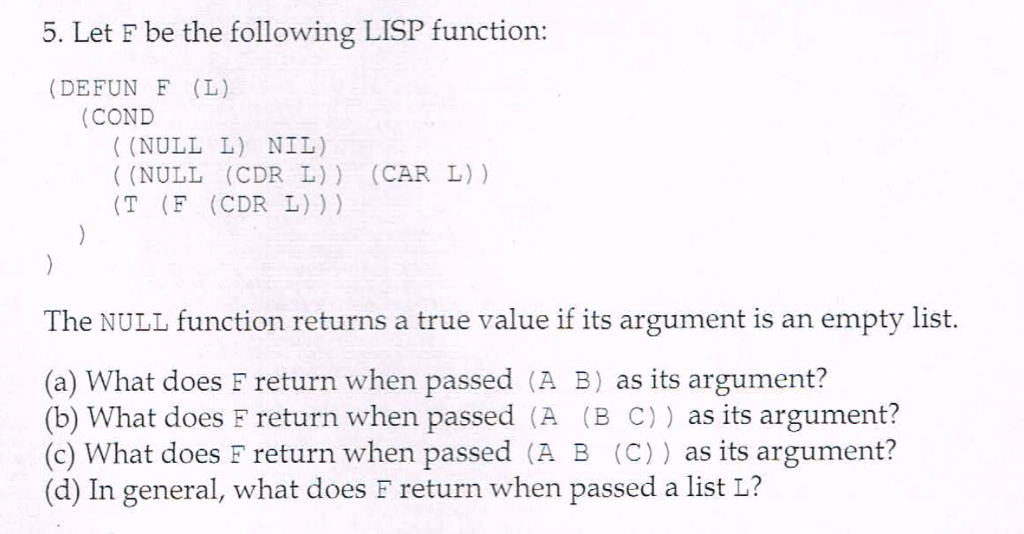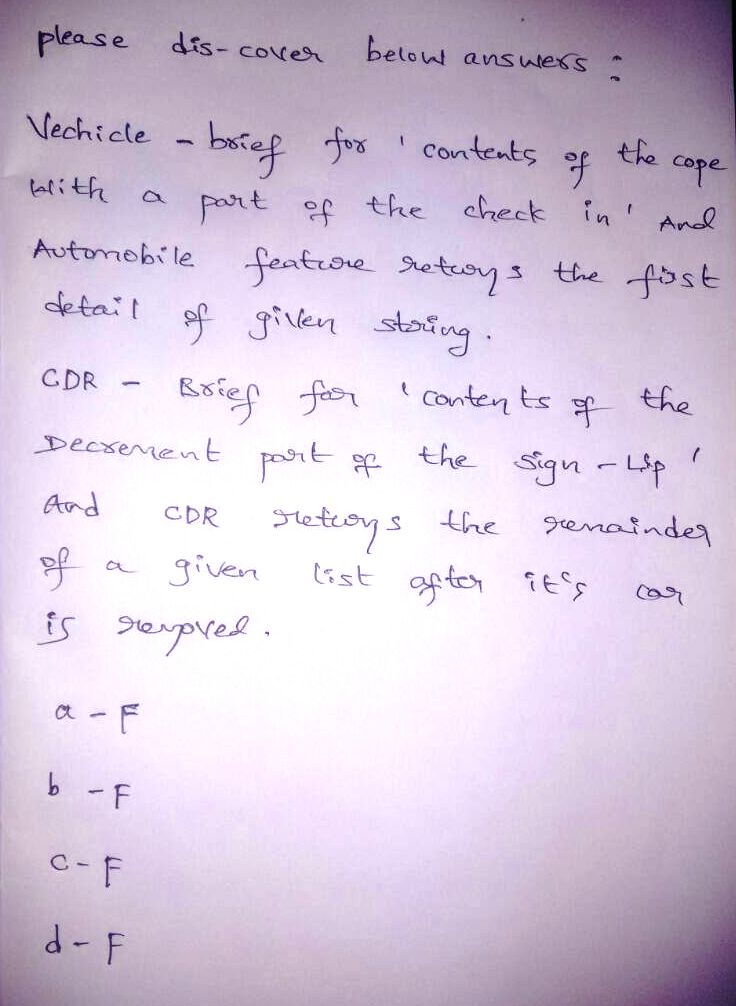# Homework Solution: Let F be the following LISP function: (DEFUN F (L) (COND ((NULL L) NIL) (NULL (…Let F be the following LISP function: (DEFUN F (L) (COND ((NULL L) NIL) (NULL (CDR L)) (CAR L)) (T (F (CDR L))) } } The NULL function returns a true value if its argument is an empty list. (a) What does F return when passed (A B) as its argument? (b) What does F return when passed (A (B C)) as its argument? (c) What does F return when passed (A B (C)) as its argument? (d) In general, what does F return when passed a list L?